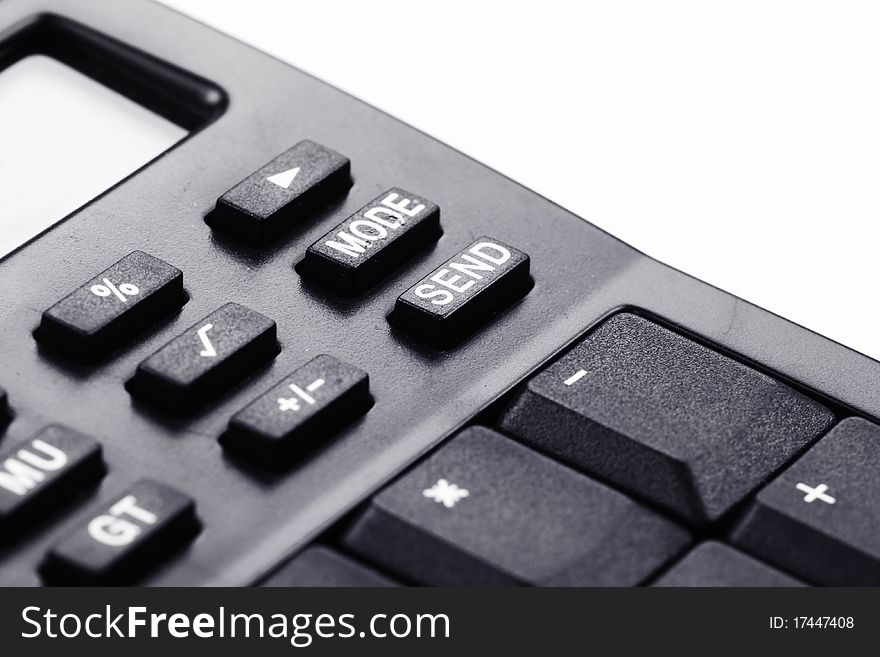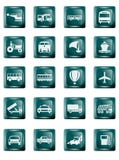Transportation Buttons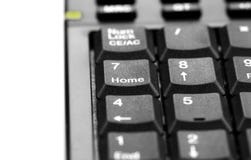Calculator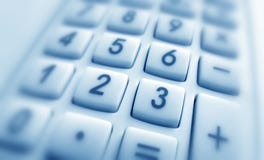Calculator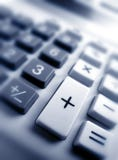Calculator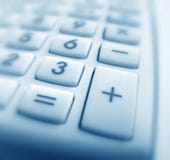Calculator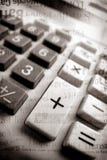Calculator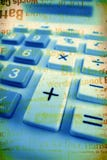Calculator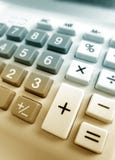Calculator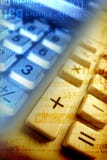Calculator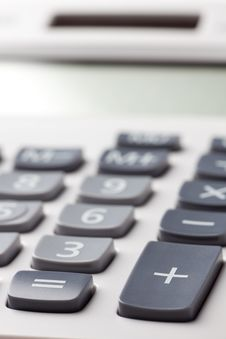Calculator Buttons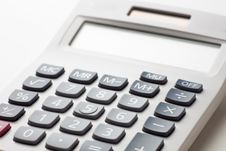Calculator Buttons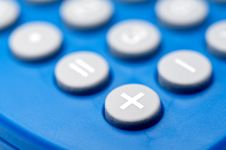Macro Of Buttons On A Calculator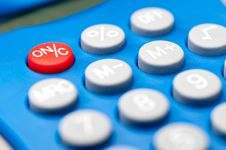Macro Of Buttons On A Calculator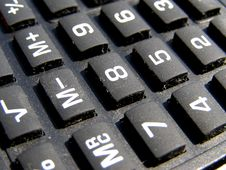Calculator Buttons Keyboard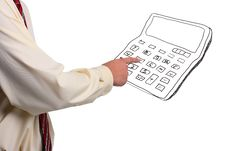Man Pressing Buttons